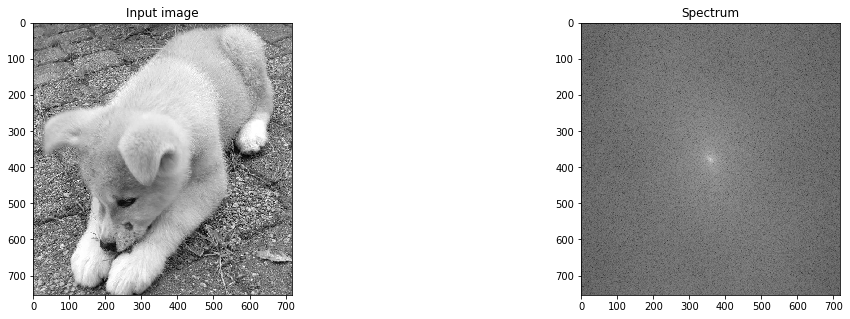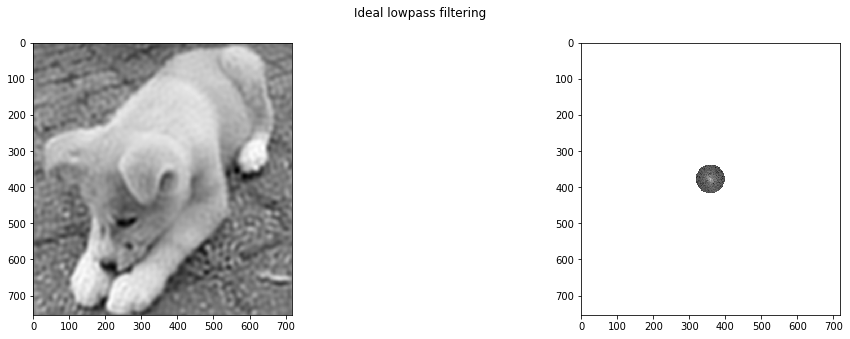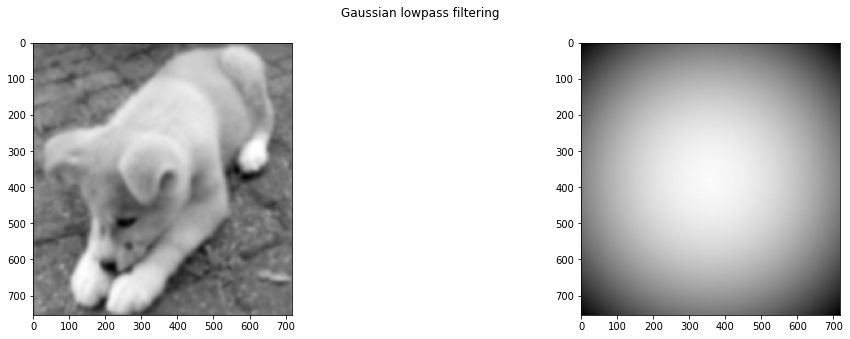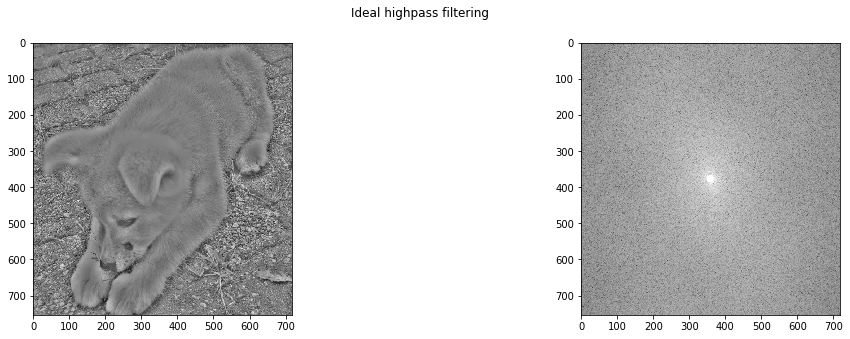# Image Filtering using Discrete Fourier Transform¶

### Examples - Astrophysics¶

Last edited: March 22nd 2018

## Introduction¶

In this notebook we will discuss the two dimensional Fourier Transform (FT) and use it to filter images. The theory behind FTs is already discussed in the notebook Discrete Fourier Transform and Fast Fourier Transform, and one dimensional FTs are used in filtering sound files in the notebook Simple Sound Filtering using Discrete Fourier Transform. If you want a deeper mathematical and physical understanding of the concepts used, we refer you to these notebooks or one of the links at the bottom of this notebook. Remember that we can interpret the Fourier Transform of the spatial domain (here the images) as the frequency domain, or frequency spectrum. For simplicity we will use images in gray-scale, but this can easily be generalised.

In :
import matplotlib.pyplot as plt
import numpy as np
import scipy.fftpack as fft
from skimage import io
import warnings
%matplotlib inline

# Taking the logarithm of zero in visualising the spectrum gives warnings,
# hence we disable them
warnings.filterwarnings("ignore")


## Fourier transforming an image¶

In order to simplify and minimize the code implementation, we create a function to visualise an image and its corresponding spectrum in the frequency domain. Note that we plot the logarithm of the absolute value, as we did in the aforementioned notebook on sound filtering.

In :
def visualise(image, spectrum, title='', title_img='', title_spec='', cmap='gray'):
""" Visualise an image and its corresponding frequency spectrum in one figure.

Parameters:
image: array-like, as in plt.imshow(). Image.
spectrum: array-like, as in plt.imshow(). Frequency spectrum.
title: string. Figure title. Optional.
title_img. string. Image subtitle. Optional.
title_spec. string. Spectrum subtitle. Optional.
cmap: ~matplotlib.colors.Colormap. Color map. Optional.
"""
plt.figure(figsize=(18, 5))
plt.subplot(121)
plt.imshow(image, cmap=cmap)
plt.title(title_img)
plt.subplot(122)
plt.imshow(np.log(abs(spectrum)), cmap=cmap)
plt.title(title_spec)
plt.suptitle(title)
plt.show()


Furthermore, we create two functions for calculating the Fourier Transform and the inverse Fourier Transform of an image. Note that these functions also shift the quadrants, such that low frequencies are collected towards the middle of the spectrum and the frequency increases with the distance from the center. This is in accordance with most tutorials online. However, it is not strictly necessary.

In :
def FFT(image):
""" Compute the 2D discrete Fast Fourier Transform of an image
and shift the zero-frequency component to the center of the spectrum.

Parameters:
image: array-like, shape (m,n), can be complex.
Returns: complex ndarray, shape (m,n). Frequency spectrum.
"""
return np.fft.fftshift(np.fft.fft2(image))

def IFFT(spectrum):
""" Shift the zero-frequency component to the edges of the spectrum and
compute the inverse 2D discrete Fast Fourier Transform of an image.

Parameters:
spectrum: array-like, shape (m,n), can be complex.
Returns: complex ndarray, shape (m,n). Spacial domain.
"""
return np.fft.ifft2(np.fft.fftshift(spectrum))


Let us take the FT of an image and plot the result.

In :
img = io.imread('https://www.numfys.net/media/notebooks/images/dog.jpg', as_grey=True)

# You may also play around with these images: (last tested March 22nd 2018)

spec = FFT(img)
visualise(img, spec, title_img='Input image', title_spec='Spectrum')## Filtering¶

There is an endless number of different filters that can be used on the frequency domain to filter the original image. We will implement filters that filter out low and high frequencies in a highpass and lowpass filter, respectively. An ideal filter and a gaussian filter is used in both cases.

Assume that the image in question has $n$ horizontal pixels ($x$-direction) and $m$ vertical pixels ($y$-direction). Let $x=0,1,...,n-1$ and $y=0,1,..,m-1$ define a square lattice $x \times y$. Now denote the frequency spectrum as $\mathcal F$, the ideal filters as $I_\text{lowpass}$ and $I_\text{highpass}$ and the gaussian filters as $G_\text{lowpass}$ and $G_\text{highpass}$, all of which are defined on the lattice $x \times y$. We define these filters such that a filtered spectrum is given by the Hadamard product (elementwise matrix product) $\mathcal F\circ I$ and $\mathcal F\circ G$.

$r=\sqrt{(x-n/2)^2+(y-m/2)^2}\geq0$ is the distance from the center of the spectrum. The filters we consider depend only on one parameter $\xi\in \mathbb{R}^+$. In this notation the ideal filters are given by

$$I_\text{lowpass}=\begin{cases} 1,& \text{for } r\leq\xi,\\ 0,& \text{for } r>\xi, \end{cases} \quad \text{and} \quad I_\text{highpass}=\begin{cases} 1,& \text{for } r\geq\xi,\\ 0,& \text{for } r<\xi, \end{cases}$$

and the gaussian filters are given by

$$G_\text{lowpass}=e^{-\xi r^2} \quad \text{and} \quad G_\text{highpass}=1-e^{-\xi r^2}.$$
In :
def filter_spectrum(spectrum, filter_type=None, val=50):
""" Filters the spectrum of an image using one of the filters defined in
the text above.

Parameters:
spectrum:    array-like, shape (m, n), can be complex. Spectrum being filtered.
filter_type: string. Filter name,
{'lowpass', 'highpass', 'gaussian_highpass', 'gaussian_lowpass'}.
val:         float. Filter parameter.
Returns: complex ndarray, shape (m, n). Filtered spectrum.
"""
n, m = np.shape(spectrum)
y, x = np.meshgrid(np.arange(1, m + 1), np.arange(1, n + 1))
R2 = ((x - n/2)**2 + (y - m/2)**2)
if (filter_type == 'lowpass'):
return spectrum*(R2 <= val**2)
elif (filter_type == 'highpass'):
return spectrum*(R2 >= val**2)
elif (filter_type == 'gaussian_highpass'):
return spectrum*(1 - np.exp(-val*R2))
elif (filter_type == 'gaussian_lowpass'):
return spectrum*np.exp(-val*R2)
elif (filter_type != None):
raise ValueError('%s is not a valid filter!' % (filter_type))
return spectrum


We now use these filters on the frequency spectrum and inverse Fourier Transforms to obtain the filtered images. Notice that the images filtered using the gaussian filters are somewhat smoother than those filtered with the ideal filters.

Play around with the code snippets!

### Lowpass¶

In :
filtered_spec = filter_spectrum(spec, 'lowpass', 40)
filtered_img = np.real(IFFT(filtered_spec))
visualise(filtered_img, filtered_spec, title='Ideal lowpass filtering')In :
filtered_spec = filter_spectrum(spec, 'gaussian_lowpass', 0.001)
filtered_img = np.real(IFFT(filtered_spec))
visualise(filtered_img, filtered_spec, title='Gaussian lowpass filtering')### Highpass¶

In :
filtered_spec = filter_spectrum(spec, 'highpass', 10)
filtered_img = np.real(IFFT(filtered_spec))
visualise(filtered_img, filtered_spec, title='Ideal highpass filtering')In :
filtered_spec = filter_spectrum(spec, 'gaussian_highpass', 0.01)
filtered_img = np.real(IFFT(filtered_spec))
visualise(filtered_img, filtered_spec, title='Gaussian highpass filtering')